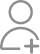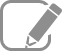Take Class 6 Tuition from the Best Tutors

•Affordable fees
•1-1 or Group class
•Flexible Timings
•Verified Tutors

Search in

# Match the following:(i) 425 × 136 = 425 × (6 + 30 + 100)(a) Commutativity under multiplication(ii) 2 × 49 × 50 = 2 × 50 × 49(b) Commutativity under addition(iii) 80 + 2005 + 20 = 80 + 20 + 2005(c) Distributivity of multiplication over addition

Learn Exercise 2.2Follow 1Answer(i) 425 × 136 = 425 × (6 + 30 + 100) Hence, (c) (ii) 2 × 49 × 50 = 2 × 50 × 49 Hence, (a) (iii) 80 + 2005 + 20 = 80 + 20 + 2005 Hence, (b)

(i) 425 × 136 = 425 × (6 + 30 + 100) [Distributivity of multiplication over addition]

Hence, (c)

(ii) 2 × 49 × 50 = 2 × 50 × 49 [Commutativity under multiplication]

Hence, (a)

(iii) 80 + 2005 + 20 = 80 + 20 + 2005 [Commutativity under addition]

Hence, (b)

Now ask question in any of the 1000+ Categories, and get Answers from Tutors and Trainers on UrbanPro.com

Find Class VI near you

Looking for Class 6 Tuition ?

Learn from the Best Tutors on UrbanPro

Are you a Tutor or Training Institute?

Join UrbanPro Today to find students near you
X

### Looking for Class 6 Tuition Classes?

The best tutors for Class 6 Tuition Classes are on UrbanPro

• Select the best Tutor
• Book & Attend a Free Demo
• Pay and start Learning### Take Class 6 Tuition with the Best Tutors

The best Tutors for Class 6 Tuition Classes are on UrbanPro